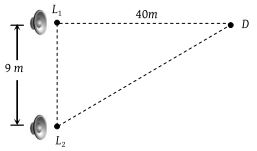An observer moves towards a stationary source of sound with a speed 1/5th of the speed of sound. The wavelength and frequency of the sound emitted are λ and f respectively. The apparent frequency and wavelength recorded by the observer are respectively :

1.

2. $f,\text{\hspace{0.17em}}1.2\lambda$

3. $0.8f,\text{\hspace{0.17em}}0.8\lambda$

4. $1.2f,\text{\hspace{0.17em}}1.2\lambda$

Concept Questions :-

Standing waves
High Yielding Test Series + Question Bank - NEET 2020

Difficulty Level:

The equation of displacement of two waves are given as ${y}_{1}=10\mathrm{sin}\left(3\pi t+\frac{\pi }{3}\right)$; ${y}_{2}=5\left(\mathrm{sin}3\pi t+\sqrt{3}\mathrm{cos}3\pi t\right)$. Then what is the ratio of their amplitudes ?

1. 1 : 2

2. 2 : 1

3. 1 : 1

4. None of these

Concept Questions :-

Wave motion
High Yielding Test Series + Question Bank - NEET 2020

Difficulty Level:

Consider ten identical sources of sound all giving the same frequency but having phase angles which are random. If the average intensity of each source is I0, the average of resultant intensity I due to all these ten sources will be :

(1) I = 100 I0

(2) I = 10 I0

(3) I = I0

(4) $I=\sqrt{10}\text{\hspace{0.17em}}{I}_{0}$

Concept Questions :-

Energy of waves
High Yielding Test Series + Question Bank - NEET 2020

Difficulty Level:

41 forks are so arranged that each produces 5 beats per sec when sounded with its near fork. If the frequency of the last fork is double the frequency of the first fork, then the frequencies of the first and last fork are respectively :

(1) 200, 400

(2) 205, 410

(3) 195, 390

(4) 100, 200

Concept Questions :-

Beats
High Yielding Test Series + Question Bank - NEET 2020

Difficulty Level:

Two identical wires have the same fundamental frequency of 400 Hz when kept under the same tension. If the tension in one wire is increased by 2%, the number of beats produced will be :

1. 4

2. 2

3. 8

4. 1

Concept Questions :-

Beats
High Yielding Test Series + Question Bank - NEET 2020

Difficulty Level:

16 tunning forks are arranged in the order of increasing frequencies. Any two successive forks give 8 beats per sec when sounded together. If the frequency of the last fork is twice the first, then the frequency of the first fork is

(1) 120

(2) 160

(3) 180

(4) 220

Concept Questions :-

Standing waves
High Yielding Test Series + Question Bank - NEET 2020

Difficulty Level:

The frequency of a stretched uniform wire under tension is in resonance with the fundamental frequency of a closed tube. If the tension in the wire is increased by 8 N, it is in resonance with the first overtone of the closed tube. The initial tension in the wire is

(1) 1 N

(2) 4 N

(3) 8 N

(4) 16 N

Concept Questions :-

Travelling wave on string
High Yielding Test Series + Question Bank - NEET 2020

Difficulty Level:

A metal wire of linear mass density of 9.8 g/m is stretched with a tension of 10 kg weight between two rigid supports 1 metre apart. The wire passes at its middle point between the poles of a permanent magnet, and it vibrates in resonance when carrying an alternating current of frequency n. The frequency n of the alternating source is :

(1) 25 Hz

(2) 50 Hz

(3) 100 Hz

(4) 200 Hz

Concept Questions :-

Travelling wave on string
High Yielding Test Series + Question Bank - NEET 2020

Difficulty Level:

An open pipe is in resonance in its 2nd harmonic with tuning fork of frequency f1. Now it is closed at one end. If the frequency of the tuning fork is increased slowly from f1 , then again a resonance is obtained with a frequency f2. If in this case the pipe vibrates in ${n}^{th}$ harmonic, then -

(1) n = 3, ${f}_{2}=\frac{3}{4}{f}_{1}$

(2) n = 3, ${f}_{2}=\frac{5}{4}{f}_{1}$

(3) n = 5, ${f}_{2}=\frac{5}{4}{f}_{1}$

(4) n = 5, ${f}_{2}=\frac{3}{4}{f}_{1}$

Concept Questions :-

Doppler effect
High Yielding Test Series + Question Bank - NEET 2020

Difficulty Level:

Two loudspeakers L1 and L2 driven by a common oscillator and amplifier, are arranged as shown. The frequency of the oscillator is gradually increased from zero and the detector at D records a series of maxima and minima. If the speed of sound is 330 ms–1 then the frequency at which the first maximum is observed is(1) 165 Hz

(2) 330 Hz

(3) 496 Hz

(4) 660 Hz

Concept Questions :-

Doppler effect